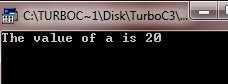# C Variables

A variable is simply a named location in memory which is used to hold a value that can be modified by the program. All variables must be declared before they can be used. Based on the data types, there can be following basic variable types:

• void
• char
• char
• int
• float

## Declare Variables in C

Here is the general form to declare a variable in C:

`type variable_name;`

Here, type must be any valid data type, and variable_name is the name of the variable. To declare more than one variable of same type, follow this general form.

`type variable_name1, variable_name2, variable_name3, ..., variable_nameN;`

Here are some variable declarations given:

```int a, b, c;
char ch1, ch2;
double bal, prof, loss;
float perc, avg;```

## Declare and Initialize Values to Variables in C

Here is the general form to declare and initialize values to variables in C:

`type variable_name = value;`

or

`type variable_name1 = value1, variable_name2 = value2, ..., variable_nameN = valueN;`

Here are some variable declaration and initialization given:

```int a = 10;
int num1 = 12, num2 = 23, num3 = 34;
float perc = 89.50;```

You can also initialize values to the variables later like this:

```int a, num1, num2, num3;
float perc;

a = 10;
num1 = 12;
num2 = 23;
num3 = 34;
perc = 89.50;```

Now let's take an example.

```#include<stdio.h>
#include<conio.h>
void main()
{
int a=10;
clrscr();

printf("The value of a is %d", a);

getch();
}```

Here is the sample output of the above C program:Here is another example.

```#include<stdio.h>
#include<conio.h>
void main()
{
int a;
clrscr();

a=20;
printf("The value of a is %d", a);

getch();
}```

Below is the sample output of the above C program:Here is one more example initializing the variables based on user input:

```#include<stdio.h>
#include<conio.h>
#include<stdlib.h>
void main()
{
int num1, num2, num3;
char ch;
clrscr();

printf("Enter the three numbers: ");
scanf("%d%d%d", &num1, &num2, &num3);
printf("Press y to see the sum of three numbers...\n");
fflush(stdin);
scanf("%c", &ch);
if(ch=='y' || ch=='Y')
{
printf("\n%d + %d + %d = %d", num1, num2, num3, num1+num2+num3);
printf("\nPress any key to exit...\n");
getch();
exit(1);
}

getch();
}```

Here is the sample run of this C program:Here the function fflush() is used to flush the buffer responsible for standard input (keyboard input). To learn more about where variables are declared or the scope rules of variables in C, then refer separate tutorial on c scope rules.

### More Examples

Here some more examples are listed, that you can go for:

Tools
Calculator